Next: 1.4 TCAD for Electromigration Up: 1.3 Empirical and Semi-Empirical Previous: 1.3.2 Resistance Increase and

As already pointed out, the lognormal distribution generally provides a good fit to EM lifetimes . The mean time to failure and the standard deviation of a lognormal distribution are calculated, respectively, by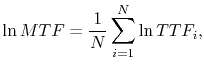(1.17)

and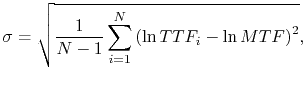(1.18)

where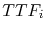is the time to failure of the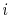-th test structure and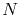is the number of test structures. The cumulative failure frequency of the-th structure is given by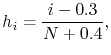(1.19)

which is then sorted in ascending order of failure times and presented in a probability plot.

The probability density function (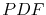) of the logonormal distribution has the form(1.20)

and the corresponding cumulative distribution function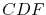,(1.21)

which represents the probability of failure until some defined time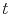. Here,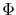is the integral of the Gaussian function,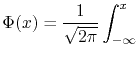exp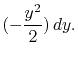(1.22)

The EM lifetime extrapolation from accelerated tests to operating conditions is based on Black's equation (1.6), and is given by [19,22]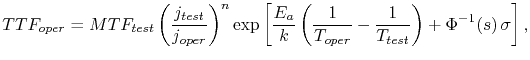(1.23)

where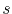is the cumulative failure percentage accepted at the real operating condition and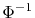is the inverse function of. The last term in (1.23) is necessary to extrapolate the 50% cumulative failure of the accelerated test to the very small failure percentiles (about 0.01%) allowed under operating conditions .

Note that this extrapolation procedure has an exponential dependence on the standard deviation of accelerated lifetimes, which might dominate the failure extrapolation over the mean time to failure. Therefore, in order to increase the interconnect lifetime at use conditions, it is necessary to increase the mean time to failure as well as to reduce the standard deviation (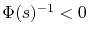for very small failure percentiles).Next: 1.4 TCAD for Electromigration Up: 1.3 Empirical and Semi-Empirical Previous: 1.3.2 Resistance Increase and

R. L. de Orio: Electromigration Modeling and Simulation